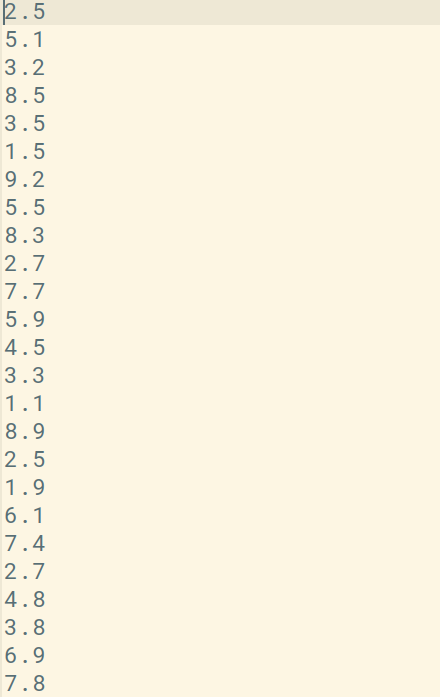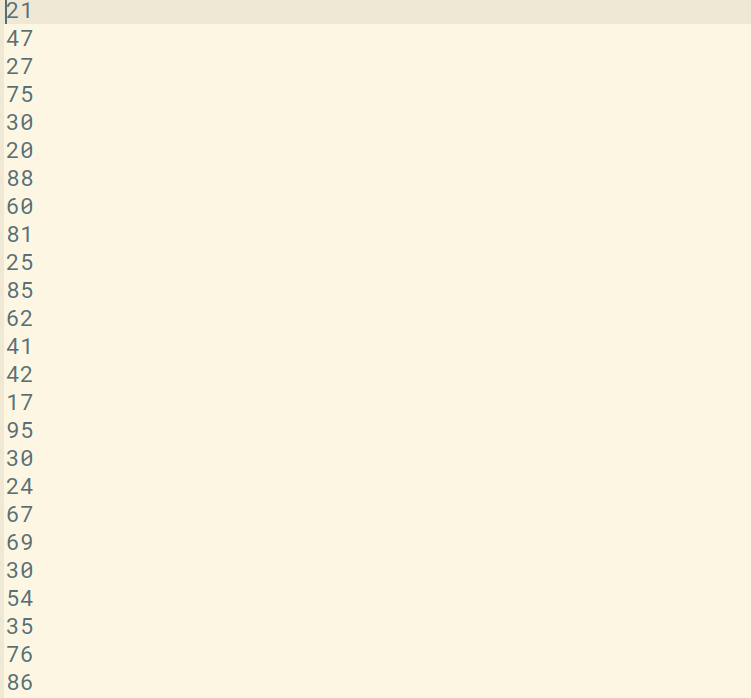GeeksforGeeks App
Open AppBrowser
Continue

# How to load and modify matrices and vectors in Octave?

In this article, we will see how to load and play with the data inside matrices and vectors in Octave. Here are some basic commands and function to regarding with matrices and vector in Octave : 1. The dimensions of the matrix : We can find the dimensions of a matrix or vector using the size() function.

## MATLAB

 `% declaring the matrix``M = [1 2 3; 4 5 6; 7 8 9];` `% dimensions of the matrix``size(M)` `% number of rows``rows = size(M, 1)` `% number of columns``cols = size(M, 2)`

Output :

```
ans =

3   3

rows =  3
cols =  3```

2. Accessing the elements of the matrix : The elements of a matrix can be accessed by passing the location of the element in parentheses. In Octave, the indexing starts from 1.

## MATLAB

 `% declaring the matrix``M = [1 2 3; 4 5 6; 7 8 9];` `% accessing the element at index (2, 3)``% i.e. 2nd row and 3rd column``M(2, 3)` `% print the 3rd row``M(3, : )` `% print the 1st column``M(:, 1)` `% print every thing from 1st and 3rd row``M([1, 3], : )`

Output :

```
ans =  6

ans =

7   8   9

ans =

1
4
7

ans =

1   2   3
7   8   9  ```

3. Longest Dimension : length() function returns the size of the longest dimension of the matrix/vector.

## MATLAB

 `% declaring the row vector``M1 = [1 2 3 4 5 6 7 8 9 10];``len_M1 = length(M1)` `% declaring the matrix``M2 = [1 2 3; 4 5 6];``len_M2 = length(M2)`

Output :

```len_M1 =  10
len_M2 =  3```

4. Loading Data : First of all let us see how to identify the directories in Octave :

## MATLAB

 `% see the present working directory``pwd  ` `% see the directory's of the folder in which you are``ls`

Output :

```ans = /home/dikshant

Now before loading the data, we need to change our present working directory to the location where our data is stored. We can do this with the cd command and then load it as follows :

## MATLAB

 `% changing the directory``cd /home/dikshant/Documents/Octave-Project` `% list the data present in this directory``ls`

Output :

`Feature.dat  target.dat`

Here we have taken the data of the scores of a student as Feature and their marks as target variable. This is the Feature.dat file which consists of 25 records of student’s study hours.This is the target.dat file which consists of 25 records of student’s marks.We can load the file with the load command in Octave, there are actually 2 ways to load the data either simply with load command or using the name of the file as a string in load(). We can use the name of the file like Feature or target to print its data.

## MATLAB

 `% loading Feature.dat``load Feature.dat ``% or load('Feature.dat')` `% loading target.dat``load target.dat  ``% or load('target.dat')` `% print Feature data``Feature` `% print target data``target` `% displaying the size of Feature file i.e. the number of data records and column``Feature_size = size(Feature)` `% displaying the size of target file i.e. the number of data records and column``target_size = size(target)`

Output :

```Feature =

2.5000
5.1000
3.2000
8.5000
3.5000
1.5000
9.2000
5.5000
8.3000
2.7000
7.7000
5.9000
4.5000
3.3000
1.1000
8.9000
2.5000
1.9000
6.1000
7.4000
2.7000
4.8000
3.8000
6.9000
7.8000

target =

21
47
27
75
30
20
88
60
81
25
85
62
41
42
17
95
30
24
67
69
30
54
35
76
86

Feature_size =

25    1

target_size =

25    1```

We can use who command for knowing the variables in our current Octave scope or whos for more a detailed description.

## MATLAB

 `% using the who command``who` `% using the whos command``whos`

Output:

```Variables in the current scope:

Feature  M        M1       M2       ans      target

Variables in the current scope:

Attr Name         Size                     Bytes  Class
==== ====         ====                     =====  =====
Feature     25x1                        200  double
M            3x3                         72  double
M1           1x10                        80  double
M2           2x3                         48  double
ans          1x2                         16  double
target      25x1                        200  double

Total is 77 elements using 616 bytes```

We can also select some of the rows from a loaded file, for example in our case 25 records data is present in Feature and target, we can create some other variable to store the trimmed data rows as shown below :

## MATLAB

 `% storing initial 5 records of Feature in var``var = Feature(1:5)` `% storing initial 5 records of target in var1``var1 = target(1:5)` `% saving the data of var in a file named modified_Feature.mat in binary format``modified_Feature.mat in binary format` `% saving the data of var1 in a file named modified_target.mat in binary format``modified_target.mat in binary format` `% saves the data in a readable format``save Feature_data.txt var -ASCII`

Output:

```var =

2.5000
5.1000
3.2000
8.5000
3.5000

var1 =

21
47
27
75
30```

5. Modifying Data :Let us now see how to modify the data of matrices and vectors.

## MATLAB

 `% declaring the matrix``M = [1 2 3; 4 5 6; 7 8 9];` `% modifying the data of 2nd column for each entry``M(:, 2) = [54; 56; 98]` `% declaring the matrix``m = [0 0 0; 0 0 0; 0 0 0];` `% modifying the data of 3rd row for each entry``m(3, 🙂 = [100; 568; 987]`

Output :

```M =

1   54    3
4   56    6
7   98    9

m =

0     0     0
0     0     0
100   568   987```

We can also append the new columns and rows in an existing matrix :

## MATLAB

 `% declaring the matrix``M = [1 2 3; 4 5 6; 7 8 9];` `% appending the new column vector to your matrix``M = [M, [20;30;40]];` `% putting all values of matrix M in a single column vector``M(:)`

Output :

```ans =

1
4
7
2
5
8
3
6
9
20
30
40```

We can also concatenate 2 different matrices :

## MATLAB

 `% declaring the matrices``a = [10 20; 30 40; 50 60];``b = [11 22; 33 44; 55 66];` `% concatenate matrix as "a" on the left and "b" on the right``c = [a b]` `% concatenate matrix as "a" on the top and "b" on the bottom``c = [a ; b]`

Output :

```c =

10   20   11   22
30   40   33   44
50   60   55   66

c =

10   20
30   40
50   60
11   22
33   44
55   66```

My Personal Notes arrow_drop_up